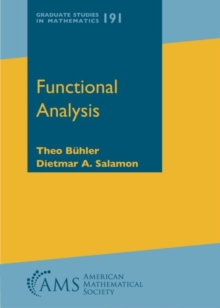# Functional Analysis Hardback

## Part of the Graduate Studies in Mathematics series

#### Description

Functional analysis is a central subject of mathematics with applications in many areas of geometry, analysis, and physics.

This book provides a comprehensive introduction to the field for graduate students and researchers.

It begins in Chapter 1 with an introduction to the necessary foundations, including the Arzela-Ascoli theorem, elementary Hilbert space theory, and the Baire Category Theorem.

Chapter 2 develops the three fundamental principles of functional analysis (uniform boundedness, open mapping theorem, Hahn-Banach theorem) and discusses reflexive spaces and the James space.

Chapter 3 introduces the weak and weak\$^*\$ topologies and includes the theorems of Banach-Alaoglu, Banach-Dieudonne, Eberlein-Smulyan, Krein-Milman, as well as an introduction to topological vector spaces and applications to ergodic theory.

Chapter 4 is devoted to Fredholm theory. It includes an introduction to the dual operator and to compact operators, and it establishes the closed image theorem.

Chapter 5 deals with the spectral theory of bounded linear operators.

It introduces complex Banach and Hilbert spaces, the continuous functional calculus for self-adjoint and normal operators, the Gelfand spectrum, spectral measures, cyclic vectors, and the spectral theorem.

Chapter 6 introduces unbounded operators and their duals.

It establishes the closed image theorem in this setting and extends the functional calculus and spectral measure to unbounded self-adjoint operators on Hilbert spaces.

Chapter 7 gives an introduction to strongly continuous semigroups and their infinitesimal generators.

It includes foundational results about the dual semigroup and analytic semigroups, an exposition of measurable functions with values in a Banach space, and a discussion of solutions to the inhomogeneous equation and their regularity properties.

The appendix establishes the equivalence of the Lemma of Zorn and the Axiom of Choice, and it contains a proof of Tychonoff's theorem.

With 10 to 20 elaborate exercises at the end of each chapter, this book can be used as a text for a one-or-two-semester course on functional analysis for beginning graduate students.

Prerequisites are first-year analysis and linear algebra, as well as some foundational material from the second-year courses on point set topology, complex analysis in one variable, and measure and integration.

#### Information

• Format: Hardback
• Pages: 472 pages
• Publisher: American Mathematical Society
• Publication Date:
• Category: Algebra
• ISBN: 9781470441906

£81.95

£81.19

on all orders

###### Pick up orders

from local bookshops

£77.50

£76.95

£93.50

£92.35

£81.95

£81.19

£97.50

£96.19

£50.50